# Split Text and Numbers

This post will guide you how to separate text and numbers from one cell in excel. How do I extract numbers from text string with formulas in Excel.

## Split Text and Numbers with Formula

If you want to split text and numbers from one cell into two different cells, you can use a formula based on the FIND function, the LEFT function or the RIGHT function and the MIN function. Just do the following steps:

#1 type the following formula in the formula box of cell C1 to get the Text part from text string in Cell A1.

`=LEFT(A1,MIN(FIND({0,1,2,3,4,5,6,7,8,9},A1&"0123456789"))-1)`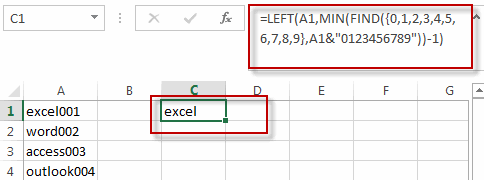#2 type the following formula in the formula box of cell D1 to get the number part from the text string in cell A1.

`=RIGHT(A1,LEN(A1)-MIN(FIND({0,1,2,3,4,5,6,7,8,9},A1&"0123456789"))+1)`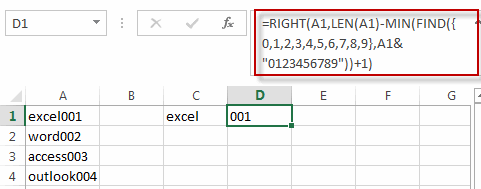3# select the cell C1 and D1, then drag the AutoFill Handle over other cell to apply those formula to split text and numbers.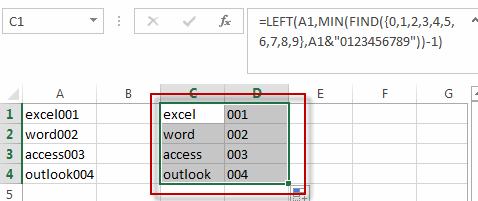## Split Text and Numbers with Flash Fill Feature

You can also use the Flash Fill function to split the text and numbers from the Cell A1, just do the following steps:

#1 type the text part of your first text string into the adjacent blank Cell B1.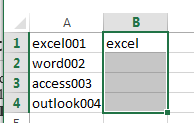#2 select the range B1:B4 where you want to fill the text part, and go to DATA tab, click Flash Fill command under Data Tools group. All text part is filled into the cells B1:B4.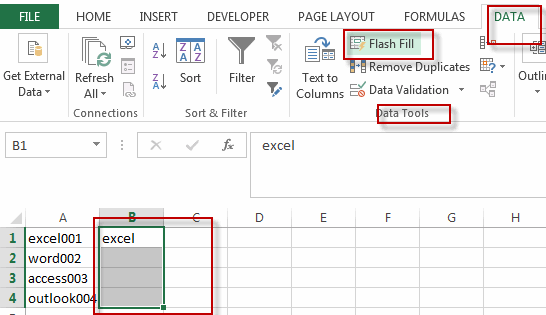#3 enter the number of your first text string into Cell C1.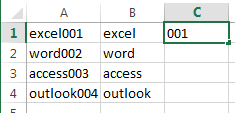#4 select the range D11:D4 where you want to fill the number part, and go to DATA tab, click Flash Fill command. All numbers are filled into the cells D1:D4.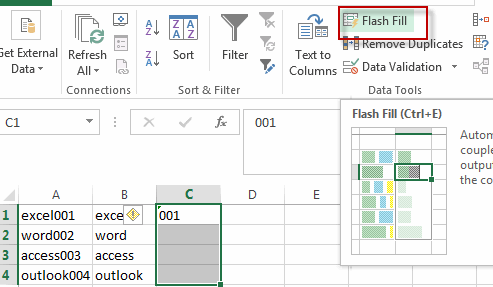#5 Lets see the result.### Related Functions

• Excel LEFT function
The Excel LEFT function returns a substring (a specified number of the characters) from a text string, starting from the leftmost character.The LEFT function is a build-in function in Microsoft Excel and it is categorized as a Text Function.The syntax of the LEFT function is as below:= LEFT(text,[num_chars])…t)…
• Excel FIND function
The Excel FIND function returns the position of the first text string (sub string) within another text string.The syntax of the FIND function is as below:= FIND(find_text, within_text,[start_num])…
• Excel MIN function
The Excel MIN function returns the smallest numeric value from the numbers that you provided. Or returns the smallest value in the array.The MIN function is a build-in function in Microsoft Excel and it is categorized as a Statistical Function.The syntax of the MIN function is as below:= MIN(num1,[num2,…numn])….
• Excel RIGHT function
The Excel RIGHT function returns a substring (a specified number of the characters) from a text string, starting from the rightmost character.The syntax of the RIGHT function is as below:= RIGHT (text,[num_chars])…
• Excel LEN function
The Excel LEN function returns the length of a text string (the number of characters in a text string).The syntax of the LEN function is as below:= LEN(text)…